3. 8 Relations and Functions:

An ordered pair is a pair of objects, whose orders are important. By convention they are represented using curve brackets () where as sets are represented using flower brackets {}.

Let A = {India, Delhi} then we note that

{India, Delhi} = {Delhi, India} (In sets, order is not important)

However in case of ordered pair

(India, Delhi)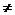(Delhi, India) (Because in an ordered pair, order is important)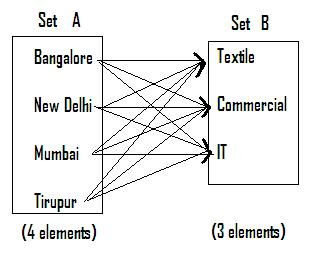Let us assume that you have been given the following question in an examination for matching.

From examination point of view there can only be three right matches. However, as it can be seen from the figure (Every element in set A is paired with every element in set B), There can be  12 possible pairs. Thus we have in all 4*3 = 12 possible pairs.

Observe that (Bangalore, IT)(IT, Bangalore)

If A and B are two given sets, the set containing all the ordered pairs where the first element is taken from A and the second element is taken from B is called ‘Cartesian product of two sets. The resulting set is denoted by A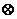B (read as A cross B).

AB = { (x,y) : x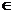A  and yB}

In the above figure it can be  observed that (Bangalore, IT)(IT, Bangalore)

Since (x,y)(y,x) ABBA

if A, B and C are three sets then the following  can be proved: that

1.      A(B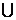C) = (AB)( AC)

2.      A(B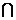C) = (AB)( AC)

3.      (B-C)A = (BA) - (CA)

4.      n(AB) = n(A)*n(B) ( n represents number of elements in the set

Some times when we have an ordered pair, it is possible to have a relationship between the elements of two sets. Some of these relations are =(is equal to), <(is less than), >(is greater than) , ||(is parallel to).

A ‘relation’ is a set of ordered pair which explains how elements of one set are related to elements of other set and the relation is normally denoted by R. Let

A = {Uttar Pradesh, Andhra Pradesh, Bihar…}

B = {Bhubaneswar, Ahmadabad, Calcutta, Chennai…}

We can have a relationship called ‘state’s capital is’.

Then, the correct relationships are {(Orissa, Bhubaneswar), (Bihar, Patna), (Gujarat, Ahmadabad),….}

Note that (Bihar, Patna)(Patna, Bihar) as the relationship changes from ‘State’s capital is’ to ‘is capital of the state’.

The set of first components of all the ordered pairs of a relation is called its domain and the set of all their second components is called range of the relation.

Let R = {(a,p), (c,q), (b,r),(b,z)} be a relation then

Domain = {a,b,c}: which is the set of all the first components of the relation R

Range  = {p,q,r,z}: which is the set of all the second components of the relation R

2.14 Problem 1 : Given that the ordered pairs (a,7) and (-4,b) belong to the relation {(x,y): 2y-3x=8}. Find the values of a and b.

Solution:

Since (a,7) satisfies the relation,

2*7 - 3*a = 814 - 3a = 8a = 2

Since (-4,b) satisfies the relation,

2b - 3*(-4) = 8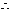2b + 12 = 8b = -2

In this example,

Domain ={2,-4}

Range  ={7,-2}

Types of relations:

‘Reflexive’ (Identity) relation: A relation R on a set is reflective, if each element of set A is related to itself. (If a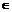A then for R to be reflective (a,a) shouldR):  The relation  ‘Greater than’ is not a Reflective relation.

‘Symmetric’ relation: A relation R on a set A is Symmetric, if for each ordered pair,(a,b)R,(b,a) alsoR (If a is related to b then for R to be Symmetric, (b,a) shouldR). The relation ‘Son of’ is not a Symmetric relation.

‘Transitive’ relation: A relation R on a set A is Transitive, if for ordered  pairs,(a,b)R,(b,c)R, then (a,c) alsoR (If a is related to b and b related c  then for R to be Transitive, (a,c) shouldR). The relation ‘Mother of’ is not a Transitive relation.

‘Equivalance’ relation: A relation R on a set A is said to be an equivalence relation if the relation is Reflective, Symmetric and Transitive. The relation ‘is congruent to’ is an equivalence relation.

A relation from set A to B is called a ‘function’ if for each element in set A there is one and only one (unique) image in set B and it is denoted by

f : A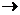B.

If (x,y) is in f (which is a relation) then we write y = f(x), where f(x) is called the value of the function at x.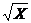Types of functions:

 Figure Function Name f : AB Property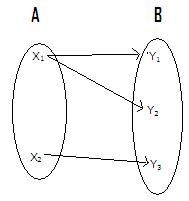This is NOT a Function   Since X1 has two images (Y1,Y2). In a function, for  one value of x there can not be more than one value of y Reason:   One element in set A is mapped onto more than one element in set B and thus it is not a function.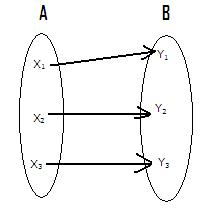One-one Function     For every value of x there is only one value of y f(X1) = Y1 f(X2) = Y2 f(X3) = Y3   For example Y = f(x) = 2x Each element of set B is the image of only one element in set A.     ================ The figure also represents One-one Onto function. (explained later)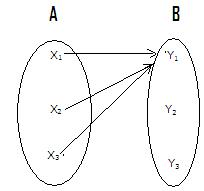Many – One Function   Y has same value for two or more different values of X f(X1) = Y1 f(X2) = Y1 f(X3) = Y1   Y = X2 is an example Two or more elements in A have the same image in B.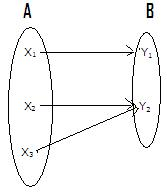Onto Function f(X1) = Y1 f(X2) = Y2 f(X3) = Y2     ================ The figure also represents Many – one onto function. If each element of set B is an image of at least one element of set A.   Thus there cannot be an element in set B which is not an image of an element in set A. ================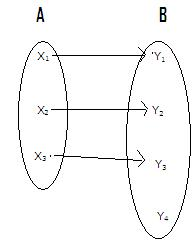Into Function   f(X1) = Y1 f(X2) = Y2 f(X3) = Y3       ================ The figure also represents One – one Into function. If there exists at least one element in set B ,which is not an image of any element in set A.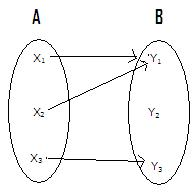Into Function   f(X1) = Y1 f(X2) = Y1 f(X3) = Y3   ================ The figure also represents Many – one Into function.

Because of the way function has been defined , we observe that f is sub set of AB and f(A)  is a sub set of B.

In fact f = {(x,f(x): xA }

2.14 Problem 2 :  Let A = {1,2,3,4,5,6}, B = {-4,-3,-2,0,1,2,3,4} and R =  {(x,y): y =2x-5: xA and YB }

Find the set R, list range and domain of R and name the  type of the function R belongs to

Solution:

Since y = 2x-5 we find that   when x = 1, y= -3(=2*1-5) then (1,-3)R

Similarly ( 2,-1),( 3,1), (4,3), (5,5), (6,7) are the other pairs which satisfy the condition y = 2x-5

We also note of these that, the element 7 in the last pair(6,7)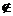B

Hence because of the definition of R (6,7)R

Domain of R = {1,2,3,4,5}

Range of R =  {-3,-1,1,3,5}

Since the element 6 in set A does not have an image in B, R is not a function.

2.14 Problem 3 :  If f(x)  = x2+2x+3 find [f(x+h) –f(x)]/h when h3

Solution:

f(x+h) = (x+h)2+2(x+h)+3 = x2+2hx + h2+2x +2h +3f(x+h)f(x) = x2+2hx + h2+2x +2h +3(x2+2x+3) = h2+2hx+2h = h(2x+h+2)[f(x+h) –f(x)]/h = 2x+h+2

If f is a function such that f : A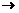B,  and  f= {(x,y), xA,yB }

Then ‘inverse of f’ is defined as f-1 = {(y,x) ; (x,y)f}

Let  is observe the following two figures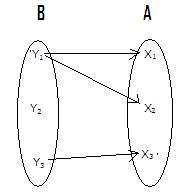FIGURE 1                                                  FIGURE   2

First figure(f : AB) is a function ( In fact it is ‘Many to one into’ ) and second figure  which is inverse of first figure f-1 : BA is not a function.

( The reason is that  y2 in B does not have a image in A)

We observe that if and only if, a function is one- one and onto, then only its inverse is a function.

As an example, note that the inverse of function f(x) = x2 is not a function. However the inverse of function f(x) = x3 is f-1(x) =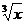Composite functions:

 If f : AB, g : BC,    then gOf : AC     is defined by   (gOf)(x) = g(f(x))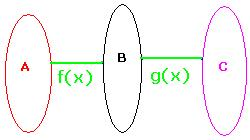2.14 Problem 4 :  If f(x)  = x+2, g(x) = x and h(x) = x2

Find gOf, hO(gOf), hOg,   and (hOg)(Of)

Solution:

{gOf}(x)  = g[f(x)]= g(x+2) =x+2

{hO (gOf)}(x) = h[gOf(x)] = h[g{f(x)}] = h[g{x+2}]= h[x+2]  = (x+2)2

{(hOg)}(x) = h[g(x)] = h(x) = x2

{hOg(Of}(x) = (hOg)(f(x)) = (hOg)(x+2) = h[g(x+2)] =h[x+2] = (x+2)2

In general we can prove that hO(gOf) = (hOg)Of

2.14 Exercise: if f(x) = 2x+1 and g(x) = x-1/2 show that fOggOf

3.8 Summary of learning

 No Points studied 1 Relations, Functions